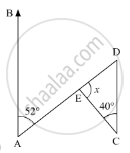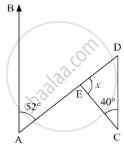Advertisement Remove all ads

# Compute the Value Of X in the Following Figure: - Mathematics

Answer in Brief

Compute the value of x in the following figure:Advertisement Remove all ads

#### Solution

In the given problem, we need to find the value of x

In the given figure, ∠BAD = 52° and  ∠DCE = 40°Here,AB || CD and AD is the transversal, so ∠EDC  and ∠BAD form a pair of alternate interior angles. Therefore, using the property, “alternate interior angles are equal”, we get,

∠EDC = ∠BAD

∠EDC = 52°

Further, applying angle sum property of the triangle

In ΔDEC

∠DEC + ∠DCE + ∠EDC = 180°

∠DEC + 40° + 52° = 180°

x + 92° = 180°

x = 180° - 92°

x = 88°

Therefore, x = 88°

Is there an error in this question or solution?
Advertisement Remove all ads

#### APPEARS IN

RD Sharma Mathematics for Class 9
Chapter 11 Triangle and its Angles
Exercise 11.2 | Q 3.3 | Page 20
Advertisement Remove all ads
Advertisement Remove all ads
Share
Notifications

View all notifications

Forgot password?
Course